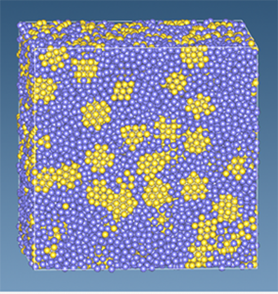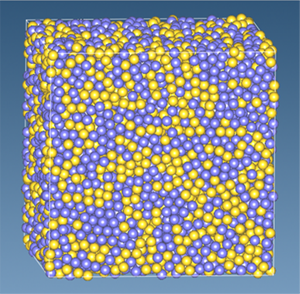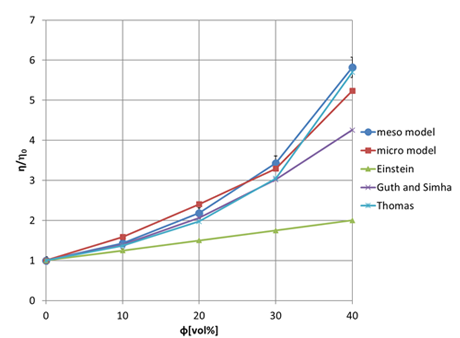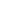Continuum Model
Mechanical / Viscosity / Viscoelasticity
Interface / Phase Separation / Particle Dispersion
Materials Science
Life Science

# [Analysis Example] Viscosity of the suspension

Fluid analysis of filler dispersion systems using the particle method (MPS).

## Objectives and Methods

Solid particles (fillers) with sizes of around 1 μm dispersed in a liquid are called suspensions, and the evaluation of their physical properties and analysis of their mechanisms are important in many industrial fields, such as inks and battery electrodes. Here, we introduce examples of viscosity evaluation.

VSOP-PS, one of the engines of J-OCTA, uses the particle method (MPS = Moving Particle Simulation (or Semi-Implicit) method) to solve the Navier-Stokes equations, the basis of fluid dynamics.
It is possible to compute solid particles in a fluid, and two models can be selected. We refer to the left side of Figure 1 as the micro model and the right side as the meso model. In the micro model, one solid particulate is modeled as “multiple yellow spheres” connected together. In the meso model, one solid particle is represented by “one yellow sphere”. The micro model can model complex solid particle shapes. On the other hand, solid particles in the meso model are more coarse-grained, resulting in the ability to calculate systems containing many solid particles.Figure 1. Suspension calculations using VSOP-PS
(left: micro model, right: meso model)

## Results

Figure 2 shows the relationship between the volume fraction of solid particles and the viscosity of the suspension.

Except for VSOP-PS (meso model and micro model), the other curves are based on well-known models, respectively.

The two VSOP-PS results are close to the Thomas model, which is often referenced in the high concentration range.

For solid particulate dispersion systems in liquids, the free version of OCTA already includes KAPSEL, which is based on the Smoothed Profile Method and provides highly accurate simulations of various phenomena in suspensions. On the other hand, VSOP-PS is being developed to target a wide range of phenomena such as droplet and evaporation phenomena, flow field in porous media, thermal coupling, etc., by taking advantage of the particle method. VSOP-PS and KAPSEL can be used separately for each target.Figure 2. Relationship between viscosity of suspension and volume fraction of solid particles